Successfully reported this slideshow.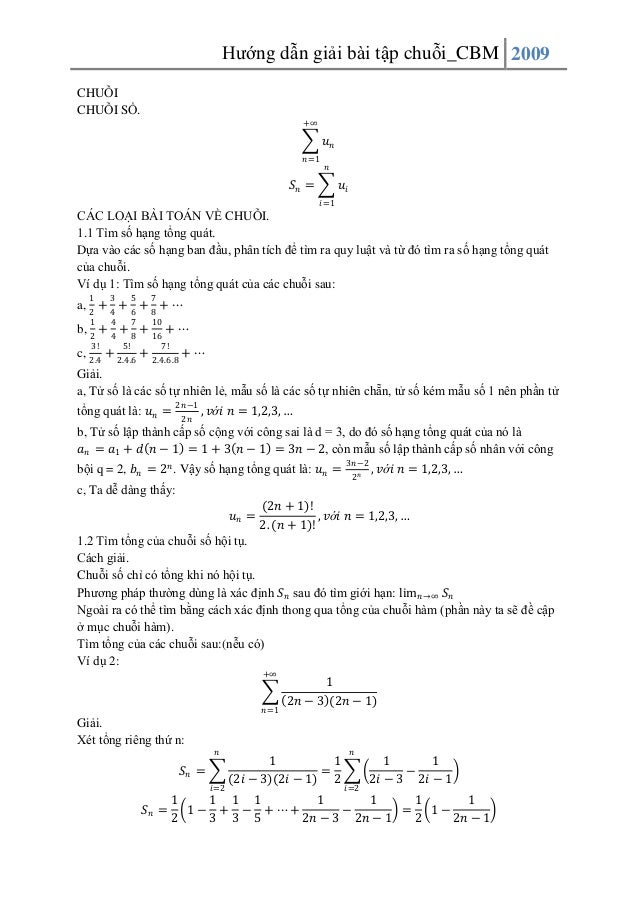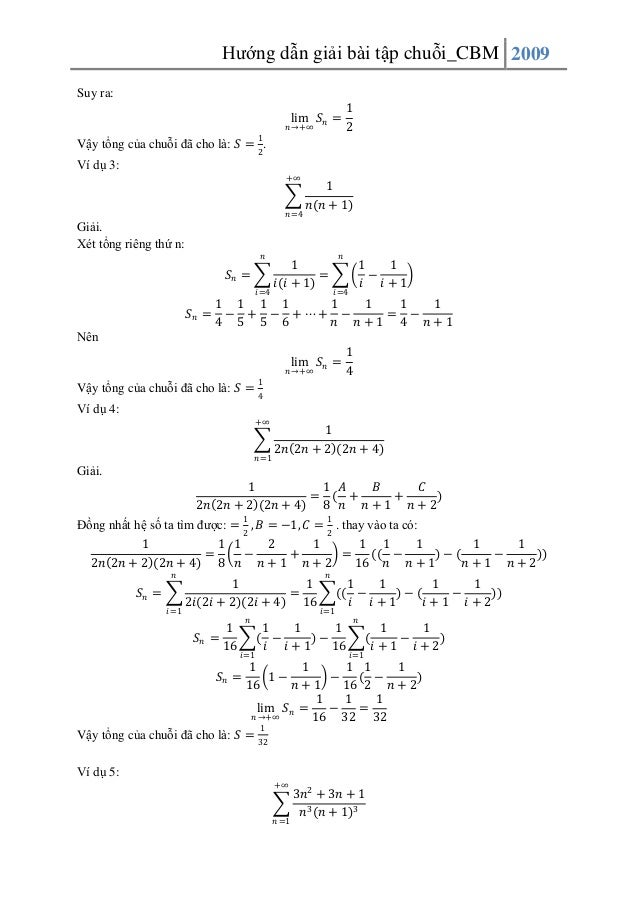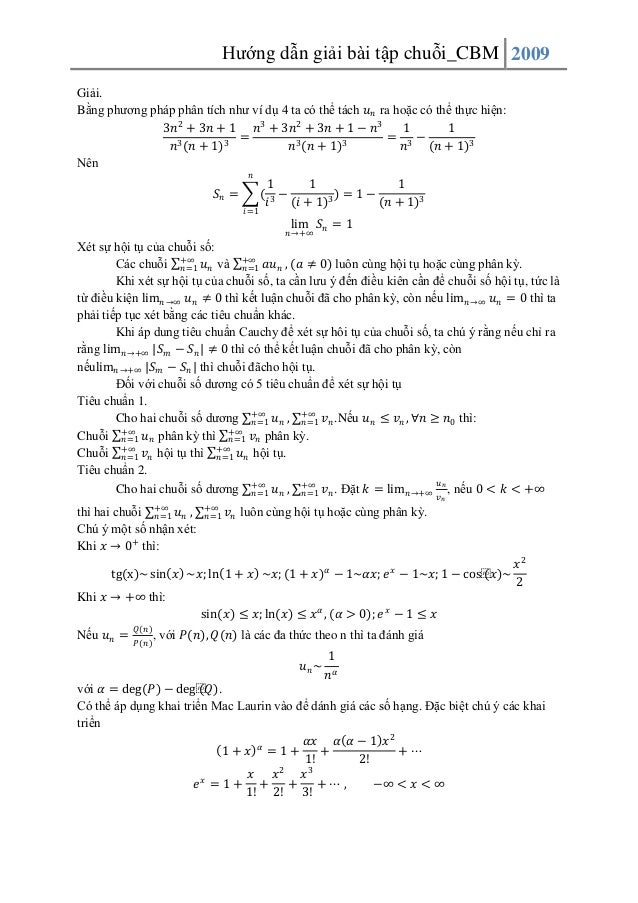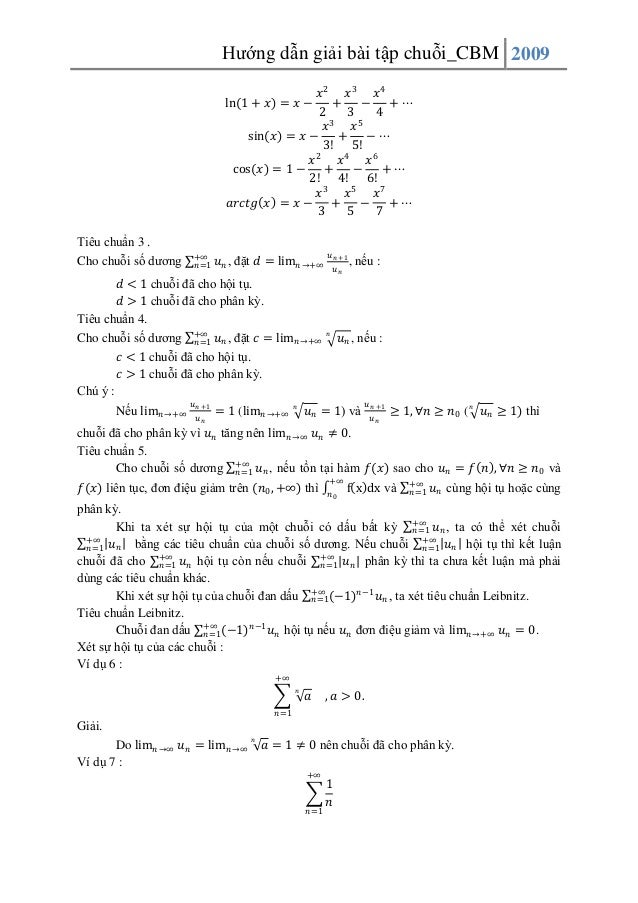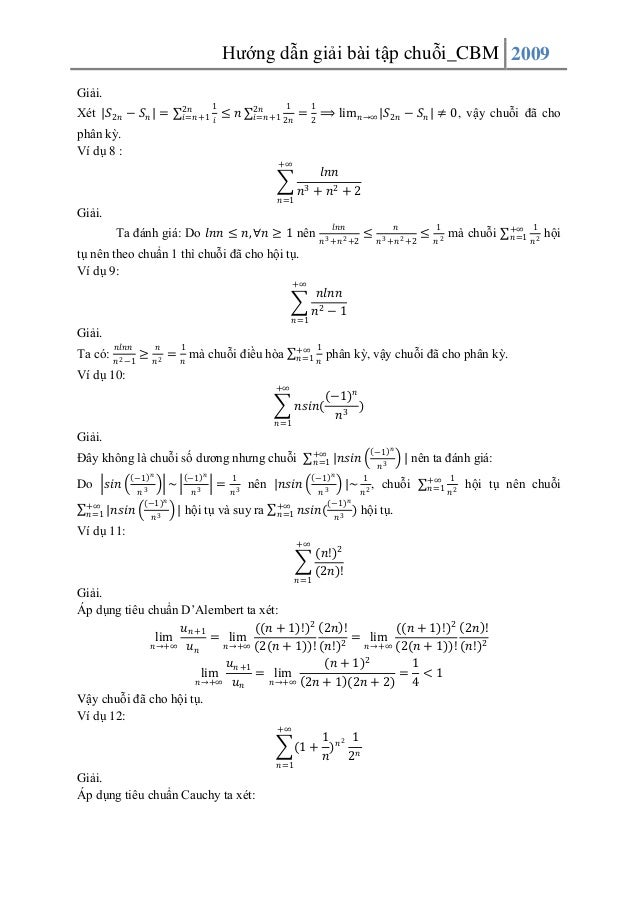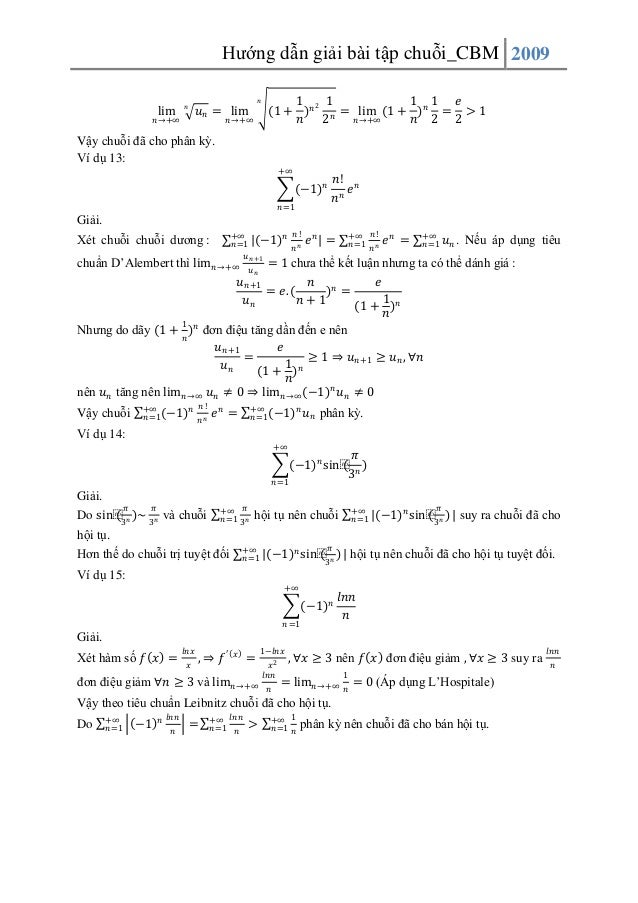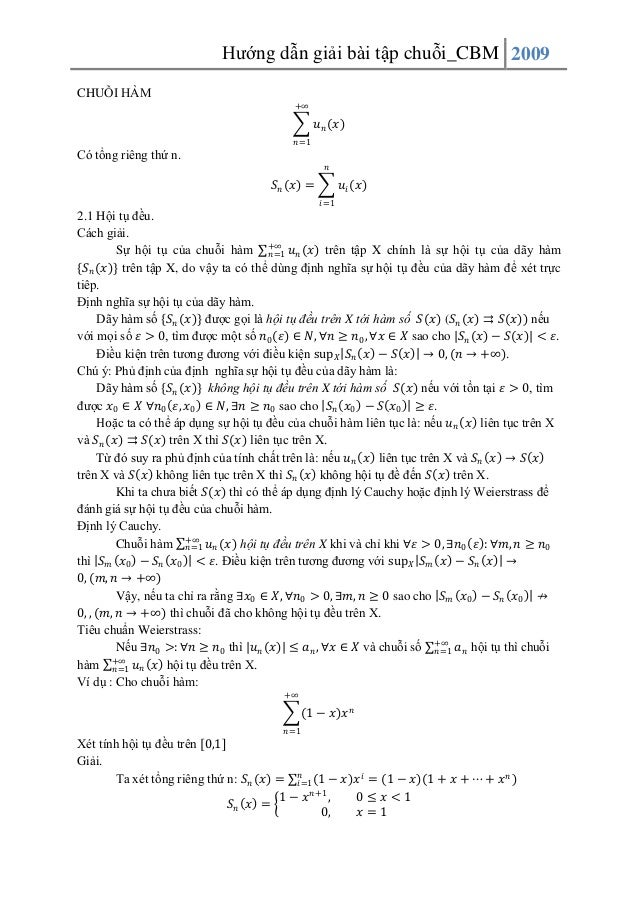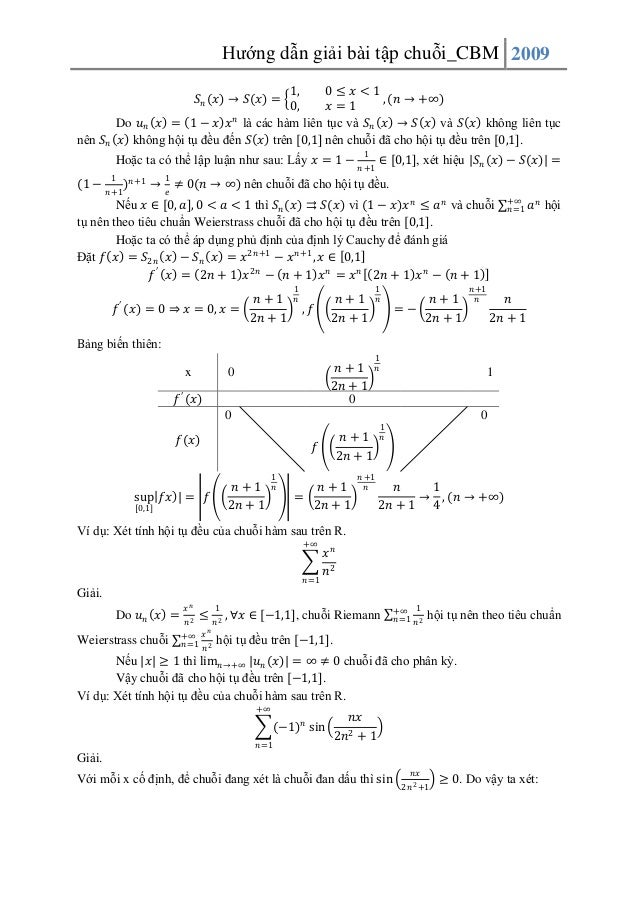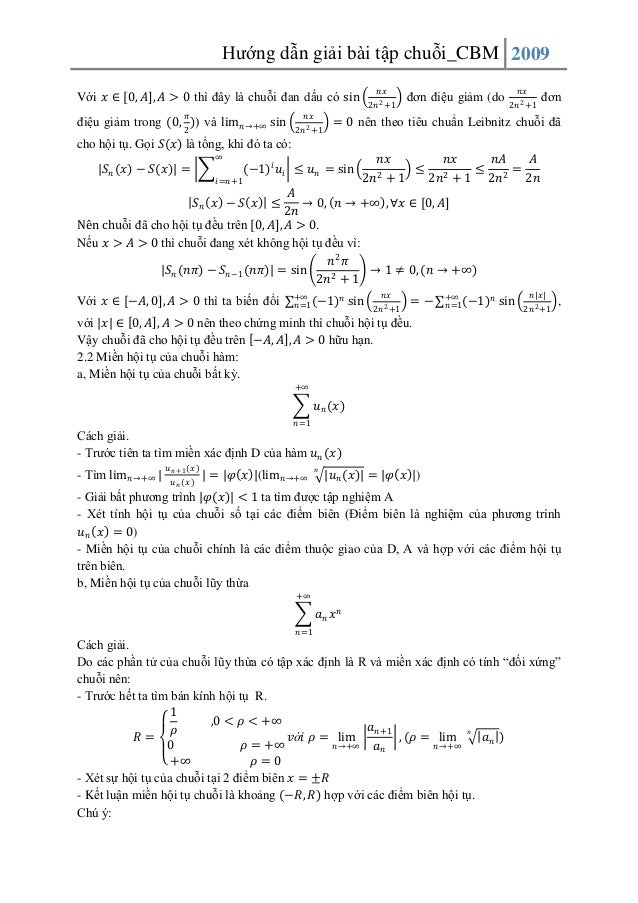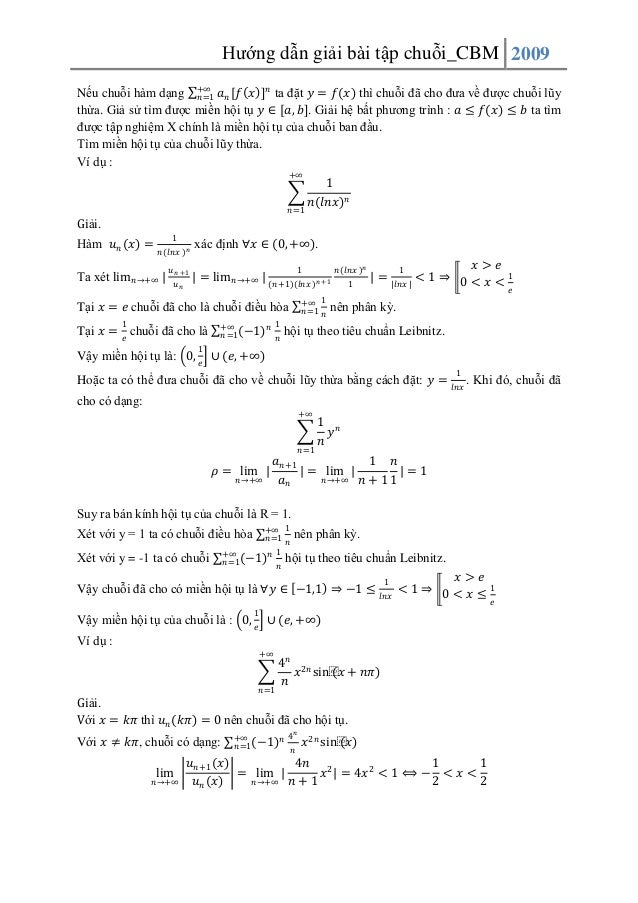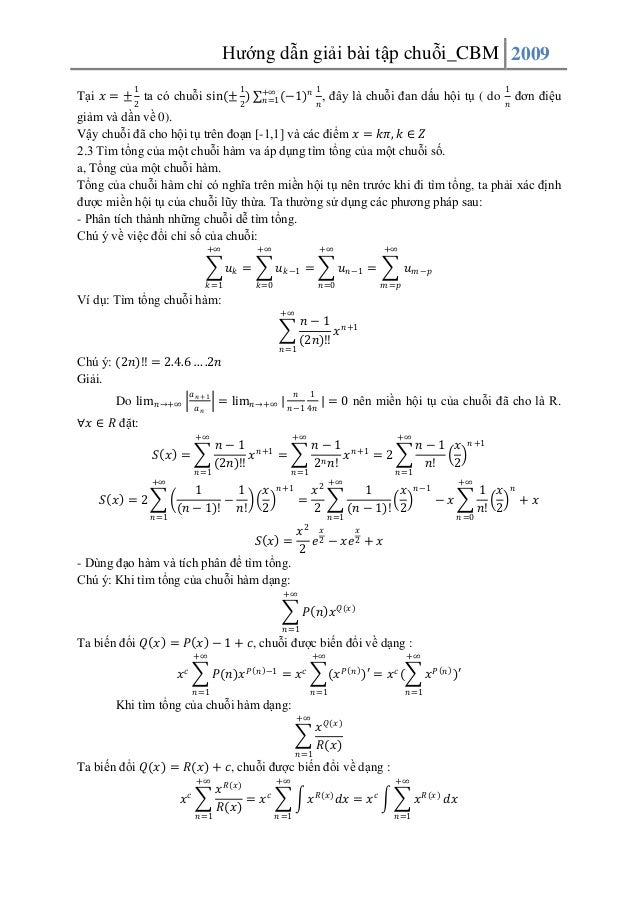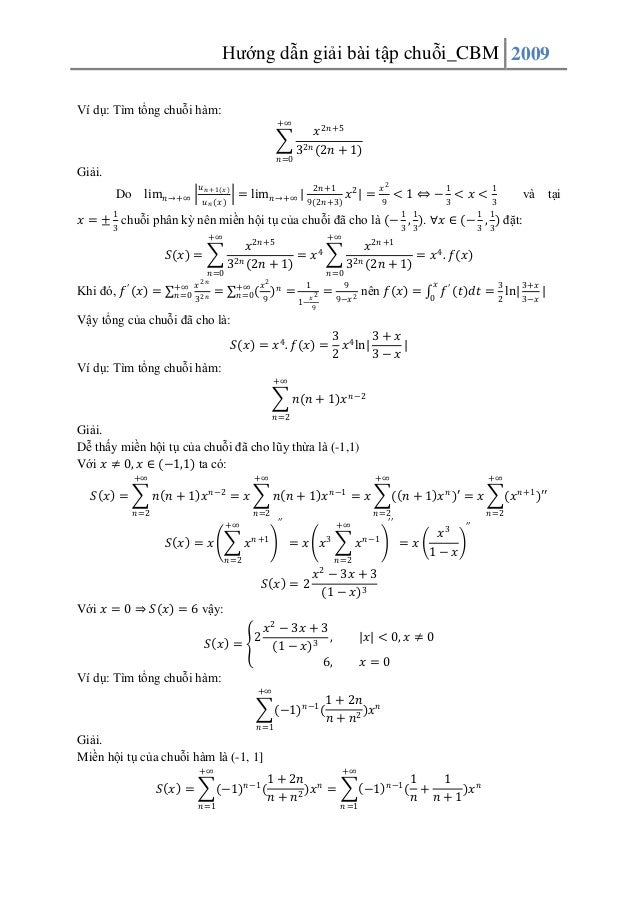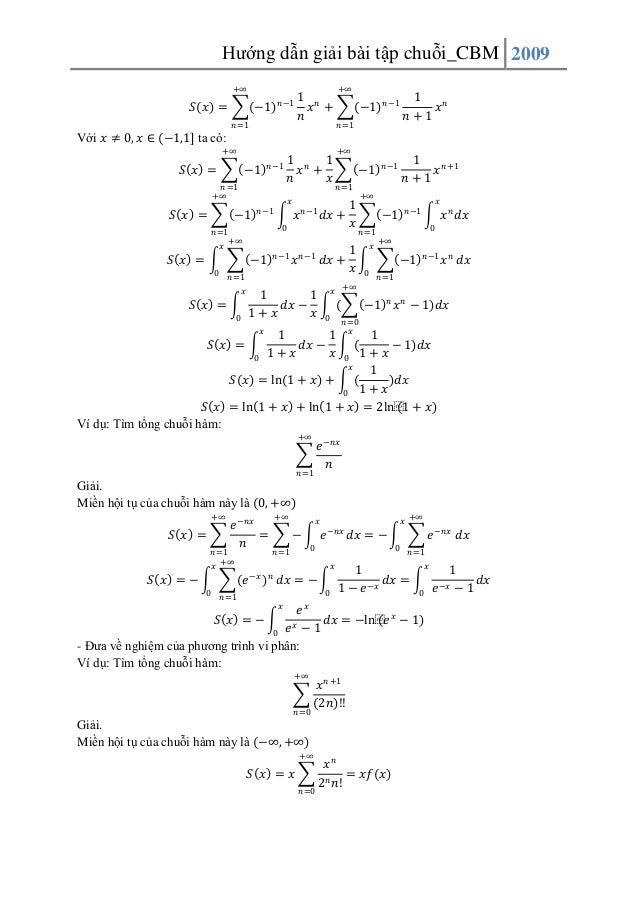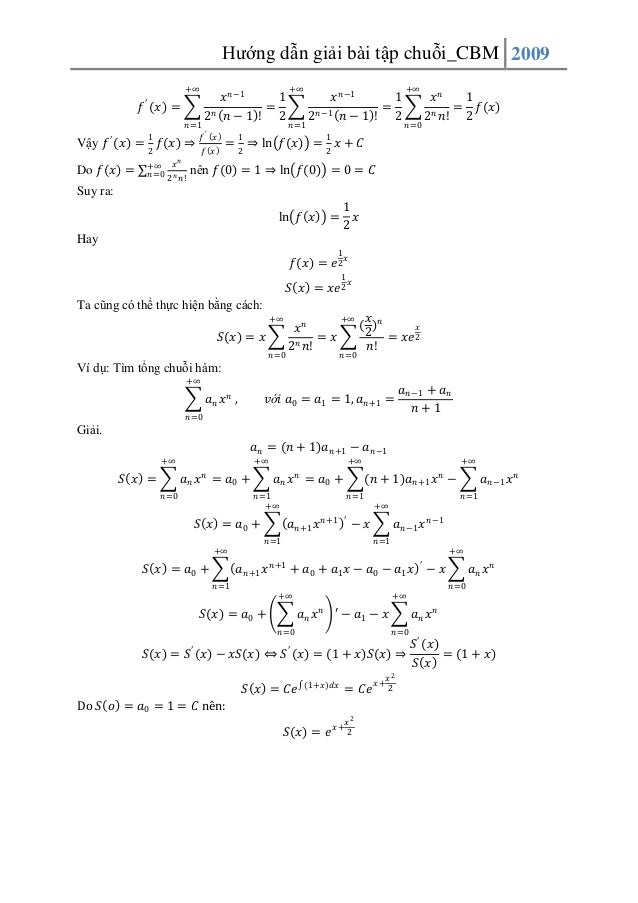Upcoming SlideShare
×

# Hướng dẫn giải bài tập chuỗi - Toán cao cấp

Hướng dẫn giải bài tập chuỗi - Toán cao cấp

• Full Name
Comment goes here.

Are you sure you want to Yes No• Be the first to comment

### Hướng dẫn giải bài tập chuỗi - Toán cao cấp

1. 1. Hướng dẫn giải bài tập chuỗi_CBM 2009 CHUỖI CHUỖI SỐ. +∞ 𝑢𝑛 𝑛=1 𝑛 𝑆𝑛 = 𝑢𝑖 𝑖=1 CÁC LOẠI BÀI TOÁN VỀ CHUỖI. 1.1 Tìm số hạng tổng quát. Dựa vào các số hạng ban đầu, phân tích để tìm ra quy luật và từ đó tìm ra số hạng tổng quát của chuỗi. Ví dụ 1: Tìm số hạng tổng quát của các chuỗi sau: 1 3 5 7 1 4 7 10 a, 2 + 4 + 6 + 8 + ⋯ b, 2 + 4 + 8 + 16 + ⋯ 3! 5! 7! c, 2.4 + 2.4.6 + 2.4.6.8 + ⋯ Giải. a, Tử số là các số tự nhiên lẻ, mẫu số là các số tự nhiên chẵn, tử số kém mẫu số 1 nên phần tử tổng quát là: 𝑢 𝑛 = 2𝑛−1 2𝑛 , 𝑣ớ𝑖 𝑛 = 1,2,3, … b, Tử số lập thành cấp số cộng với công sai là d = 3, do đó số hạng tổng quát của nó là 𝑎 𝑛 = 𝑎1 + 𝑑 𝑛 − 1 = 1 + 3 𝑛 − 1 = 3𝑛 − 2, còn mẫu số lập thành cấp số nhân với công bội q = 2, 𝑏 𝑛 = 2 𝑛 . Vậy số hạng tổng quát là: 𝑢 𝑛 = 3𝑛−2 2𝑛 , 𝑣ớ𝑖 𝑛 = 1,2,3, … c, Ta dễ dàng thấy: 𝑢𝑛 = (2𝑛 + 1)! , 𝑣ớ𝑖 𝑛 = 1,2,3, … 2. (𝑛 + 1)! 1.2 Tìm tổng của chuỗi số hội tụ. Cách giải. Chuỗi số chỉ có tổng khi nó hội tụ. Phương pháp thường dùng là xác định 𝑆 𝑛 sau đó tìm giới hạn: lim 𝑛→∞ 𝑆 𝑛 Ngoài ra có thể tìm bằng cách xác định thong qua tổng của chuỗi hàm (phần này ta sẽ đề cập ở mục chuỗi hàm). Tìm tổng của các chuỗi sau:(nễu có) Ví dụ 2: +∞ 𝑛=1 Giải. Xét tổng riêng thứ n: 𝑛 𝑆𝑛 = 𝑖=2 1 1 2𝑛 − 3 (2𝑛 − 1) 1 = 2𝑖 − 3 2𝑖 − 1 2 𝑛 𝑖=2 1 1 − 2𝑖 − 3 2𝑖 − 1 1 1 1 1 1 1 1 1 𝑆𝑛 = 1 − + − + ⋯+ − = 1− 2 3 3 5 2𝑛 − 3 2𝑛 − 1 2 2𝑛 − 1
2. 2. Hướng dẫn giải bài tập chuỗi_CBM 2009 Suy ra: lim 𝑆 𝑛 = 1 𝑛→+∞ 1 2 Vậy tổng của chuỗi đã cho là: 𝑆 = 2. Ví dụ 3: +∞ 𝑛 =4 1 𝑛(𝑛 + 1) Giải. Xét tổng riêng thứ n: 𝑛 𝑆𝑛 = 𝑖=4 1 = 𝑖(𝑖 + 1) 𝑛 1 1 − 𝑖 𝑖+1 𝑖=4 1 1 1 1 1 1 1 1 𝑆𝑛 = − + − + ⋯+ − = − 4 5 5 6 𝑛 𝑛+1 4 𝑛+1 Nên lim 𝑆 𝑛 = 1 𝑛→+∞ 1 4 Vậy tổng của chuỗi đã cho là: 𝑆 = 4 Ví dụ 4: +∞ 𝑛=1 1 2𝑛 2𝑛 + 2 (2𝑛 + 4) Giải. 1 1 𝐴 𝐵 𝐶 = ( + + ) 2𝑛 2𝑛 + 2 (2𝑛 + 4) 8 𝑛 𝑛+1 𝑛+2 1 1 Đồng nhất hệ số ta tìm được: = 2 , 𝐵 = −1, 𝐶 = 2 . thay vào ta có: 1 1 1 2 1 1 1 1 1 1 = − + = (( − )−( − )) 2𝑛 2𝑛 + 2 (2𝑛 + 4) 8 𝑛 𝑛+1 𝑛+2 16 𝑛 𝑛 + 1 𝑛+1 𝑛+2 𝑛 𝑆𝑛 = 𝑖=1 1 1 = 2𝑖 2𝑖 + 2 (2𝑖 + 4) 16 1 𝑆𝑛 = 16 𝑛 𝑖=1 𝑛 𝑖=1 1 1 1 1 (( − )−( − )) 𝑖 𝑖+1 𝑖+1 𝑖+2 1 1 1 ( − )− 𝑖 𝑖+1 16 𝑛 ( 𝑖=1 1 1 − ) 𝑖+1 𝑖+2 1 1 1 1 1 𝑆𝑛 = 1− − ( − ) 16 𝑛+1 16 2 𝑛 + 2 1 1 1 lim 𝑆 𝑛 = − = 𝑛 →+∞ 16 32 32 1 Vậy tổng của chuỗi đã cho là: 𝑆 = 32 Ví dụ 5: +∞ 𝑛 =1 3𝑛2 + 3𝑛 + 1 𝑛3 (𝑛 + 1)3
3. 3. Hướng dẫn giải bài tập chuỗi_CBM 2009 Giải. Bằng phương pháp phân tích như ví dụ 4 ta có thể tách 𝑢 𝑛 ra hoặc có thể thực hiện: 3𝑛2 + 3𝑛 + 1 𝑛3 + 3𝑛2 + 3𝑛 + 1 − 𝑛3 1 1 = = 3− 𝑛3 (𝑛 + 1)3 𝑛3 (𝑛 + 1)3 𝑛 (𝑛 + 1)3 Nên 𝑛 𝑆𝑛 = ( 𝑖=1 1 1 1 − )= 1− 𝑖 3 (𝑖 + 1)3 (𝑛 + 1)3 lim 𝑆 𝑛 = 1 𝑛→+∞ Xét sự hội tụ của chuỗi số: Các chuỗi +∞ 𝑢 𝑛 và +∞ 𝑎𝑢 𝑛 , (𝑎 ≠ 0) luôn cùng hội tụ hoặc cùng phân kỳ. 𝑛=1 𝑛=1 Khi xét sự hội tụ của chuỗi số, ta cần lưu ý đến điều kiên cần để chuỗi số hội tụ, tức là từ điều kiện lim 𝑛 →∞ 𝑢 𝑛 ≠ 0 thì kết luận chuỗi đã cho phân kỳ, còn nếu lim 𝑛→∞ 𝑢 𝑛 = 0 thì ta phải tiếp tục xét bằng các tiêu chuẩn khác. Khi áp dung tiêu chuẩn Cauchy để xét sự hôi tụ của chuỗi số, ta chú ý rằng nếu chỉ ra rằng lim 𝑛→+∞ |𝑆 𝑚 − 𝑆 𝑛 | ≠ 0 thì có thể kết luận chuỗi đã cho phân kỳ, còn nếulim 𝑛 →+∞ |𝑆 𝑚 − 𝑆 𝑛 | thì chuỗi đãcho hội tụ. Đối với chuỗi số dương có 5 tiêu chuẩn để xét sự hội tụ Tiêu chuẩn 1. Cho hai chuỗi số dương +∞ 𝑢 𝑛 , +∞ 𝑣 𝑛 .Nếu 𝑢 𝑛 ≤ 𝑣 𝑛 , ∀𝑛 ≥ 𝑛0 thì: 𝑛=1 𝑛=1 Chuỗi +∞ 𝑢 𝑛 phân kỳ thì +∞ 𝑣 𝑛 phân kỳ. 𝑛 =1 𝑛=1 Chuỗi +∞ 𝑣 𝑛 hội tụ thì +∞ 𝑢 𝑛 hội tụ. 𝑛 =1 𝑛=1 Tiêu chuẩn 2. 𝑢 Cho hai chuỗi số dương +∞ 𝑢 𝑛 , +∞ 𝑣 𝑛 . Đặt 𝑘 = lim 𝑛→+∞ 𝑣 𝑛 , nếu 0 < 𝑘 < +∞ 𝑛=1 𝑛=1 +∞ 𝑛=1 thì hai chuỗi 𝑢𝑛 , Chú ý một số nhận xét: Khi 𝑥 → 0+ thì: +∞ 𝑛=1 𝑛 𝑣 𝑛 luôn cùng hội tụ hoặc cùng phân kỳ. tg(x)~ sin 𝑥 ~𝑥; ln 1 + 𝑥 ~𝑥; (1 + 𝑥) 𝛼 − 1~𝛼𝑥; 𝑒 𝑥 − 1~𝑥; 1 − cos⁡ (𝑥)~ Khi 𝑥 → +∞ thì: 𝑥2 2 sin 𝑥 ≤ 𝑥; ln 𝑥 ≤ 𝑥 𝛼 , 𝛼 > 0 ; 𝑒 𝑥 − 1 ≤ 𝑥 Nếu 𝑢 𝑛 = 𝑄(𝑛 ) 𝑃(𝑛 ) , với 𝑃 𝑛 , 𝑄(𝑛) là các đa thức theo n thì ta đánh giá 𝑢 𝑛~ 1 𝑛𝛼 với 𝛼 = deg 𝑃 − deg⁡ (𝑄). Có thể áp dụng khai triển Mac Laurin vào để dánh giá các số hạng. Đặc biệt chú ý các khai triển 𝛼𝑥 𝛼 𝛼 − 1 𝑥2 𝛼 1+ 𝑥 =1+ + +⋯ 1! 2! 𝑥 𝑥2 𝑥3 𝑒𝑥 = 1+ + + +⋯ , −∞ < 𝑥 < ∞ 1! 2! 3!
4. 4. Hướng dẫn giải bài tập chuỗi_CBM 2009 𝑥2 𝑥3 𝑥4 + − +⋯ 2 3 4 3 5 𝑥 𝑥 sin 𝑥 = 𝑥 − + − ⋯ 3! 5! 2 𝑥 𝑥4 𝑥6 cos 𝑥 = 1 − + − + ⋯ 2! 4! 6! 𝑥3 𝑥5 𝑥7 𝑎𝑟𝑐𝑡𝑔 𝑥 = 𝑥 − + − + ⋯ 3 5 7 ln 1 + 𝑥 = 𝑥 − Tiêu chuẩn 3 . Cho chuỗi số dương +∞ 𝑛=1 𝑢 𝑛 , đặt 𝑑 = lim 𝑛 →+∞ 𝑢 𝑛 +1 , 𝑢𝑛 nếu : 𝑑 < 1 chuỗi đã cho hội tụ. 𝑑 > 1 chuỗi đã cho phân kỳ. Tiêu chuẩn 4. Cho chuỗi số dương +∞ 𝑢 𝑛 , đặt 𝑐 = lim 𝑛 →+∞ 𝑛 𝑢 𝑛 , nếu : 𝑛=1 𝑐 < 1 chuỗi đã cho hội tụ. 𝑐 > 1 chuỗi đã cho phân kỳ. Chú ý : 𝑢 𝑢 Nếu lim 𝑛→+∞ 𝑢𝑛 +1 = 1 (lim 𝑛 →+∞ 𝑛 𝑢 𝑛 = 1) và 𝑢𝑛 +1 ≥ 1, ∀𝑛 ≥ 𝑛0 ( 𝑛 𝑢 𝑛 ≥ 1) thì 𝑛 𝑛 chuỗi đã cho phân kỳ vì 𝑢 𝑛 tăng nên lim 𝑛 →∞ 𝑢 𝑛 ≠ 0. Tiêu chuẩn 5. Cho chuỗi số dương +∞ 𝑢 𝑛 , nếu tồn tại hàm 𝑓(𝑥) sao cho 𝑢 𝑛 = 𝑓 𝑛 , ∀𝑛 ≥ 𝑛0 và 𝑛=1 𝑓(𝑥) liên tục, đơn điệu giảm trên (𝑛0 , +∞) thì +∞ f 𝑛0 x dx và +∞ 𝑛=1 𝑢 𝑛 cùng hội tụ hoặc cùng phân kỳ. Khi ta xét sự hội tụ của một chuỗi có dấu bất kỳ +∞ 𝑢 𝑛 , ta có thể xét chuỗi 𝑛 =1 +∞ 𝑢 𝑛 bằng các tiêu chuẩn của chuỗi số dương. Nếu chuỗi +∞ 𝑢 𝑛 hội tụ thì kết luận 𝑛=1 𝑛=1 chuỗi đã cho +∞ 𝑢 𝑛 hội tụ còn nếu chuỗi +∞ 𝑢 𝑛 phân kỳ thì ta chưa kết luận mà phải 𝑛=1 𝑛=1 dùng các tiêu chuẩn khác. Khi xét sự hội tụ của chuỗi đan dấu +∞ (−1) 𝑛−1 𝑢 𝑛 , ta xét tiêu chuẩn Leibnitz. 𝑛=1 Tiêu chuẩn Leibnitz. Chuỗi đan dấu +∞ (−1) 𝑛−1 𝑢 𝑛 hội tụ nếu 𝑢 𝑛 đơn điệu giảm và lim 𝑛→+∞ 𝑢 𝑛 = 0. 𝑛=1 Xét sự hội tụ của các chuỗi : Ví dụ 6 : +∞ 𝑛 𝑎 , 𝑎 > 0. 𝑛=1 Giải. Do lim 𝑛 →∞ 𝑢 𝑛 = lim 𝑛 →∞ Ví dụ 7 : 𝑛 𝑎 = 1 ≠ 0 nên chuỗi đã cho phân kỳ. +∞ 𝑛=1 1 𝑛
5. 5. Hướng dẫn giải bài tập chuỗi_CBM 2009 Giải. Xét 𝑆2𝑛 − 𝑆 𝑛 = 1 2𝑛 𝑖=𝑛+1 𝑖 ≤ 𝑛 1 2𝑛 𝑖=𝑛+1 2𝑛 1 = 2 ⟹ lim 𝑛→∞ 𝑆2𝑛 − 𝑆 𝑛 ≠ 0, vậy chuỗi đã cho phân kỳ. Ví dụ 8 : +∞ 𝑛3 𝑛=1 𝑙𝑛𝑛 + 𝑛2 + 2 Giải. 𝑙𝑛𝑛 Ta đánh giá: Do 𝑙𝑛𝑛 ≤ 𝑛, ∀𝑛 ≥ 1 nên 𝑛 3 +𝑛 2 +2 ≤ 𝑛 𝑛 3 +𝑛 2 +2 ≤ 1 𝑛2 mà chuỗi +∞ 1 𝑛 =1 𝑛 2 hội tụ nên theo chuẩn 1 thì chuỗi đã cho hội tụ. Ví dụ 9: +∞ 𝑛=1 𝑛𝑙𝑛𝑛 −1 𝑛2 Giải. Ta có: 𝑛𝑙𝑛𝑛 𝑛 2 −1 𝑛 ≥ 𝑛2 = 1 𝑛 +∞ 1 𝑛=1 𝑛 mà chuỗi điều hòa phân kỳ, vậy chuỗi đã cho phân kỳ. Ví dụ 10: +∞ 𝑛𝑠𝑖𝑛( 𝑛 =1 −1 𝑛 ) 𝑛3 Giải. Do 𝑠𝑖𝑛 −1 𝑛 +∞ 𝑛=1 |𝑛𝑠𝑖𝑛 𝑛3 −1 𝑛 𝑛3 ~ −1 𝑛 𝑛3 = 1 𝑛3 nên |𝑛𝑠𝑖𝑛 | hội tụ và suy ra +∞ 𝑛=1 −1 𝑛 +∞ 𝑛=1 |𝑛𝑠𝑖𝑛 Đây không là chuỗi số dương nhưng chuỗi −1 𝑛 𝑛3 𝑛𝑠𝑖𝑛( |~ −1 𝑛 𝑛3 𝑛3 1 𝑛2 | nên ta đánh giá: , chuỗi +∞ 1 𝑛=1 𝑛 2 hội tụ nên chuỗi ) hội tụ. Ví dụ 11: +∞ 𝑛=1 (𝑛!)2 2𝑛 ! Giải. Áp dụng tiêu chuẩn D’Alembert ta xét: 𝑢 𝑛 +1 ((𝑛 + 1)!)2 2𝑛 ! ((𝑛 + 1)!)2 2𝑛 ! lim = lim = lim 𝑛→+∞ 𝑢 𝑛 𝑛→+∞ 2(𝑛 + 1) ! (𝑛!)2 𝑛 →+∞ 2(𝑛 + 1) ! (𝑛!)2 𝑢 𝑛 +1 (𝑛 + 1)2 1 lim = lim = <1 𝑛→+∞ 𝑢 𝑛 𝑛 →+∞ 2𝑛 + 1 (2𝑛 + 2) 4 Vậy chuỗi đã cho hội tụ. Ví dụ 12: +∞ 𝑛=1 Giải. Áp dụng tiêu chuẩn Cauchy ta xét: 1 2 1 (1 + ) 𝑛 𝑛 𝑛 2
6. 6. Hướng dẫn giải bài tập chuỗi_CBM 2009 lim 𝑛 𝑛 𝑢 𝑛 = lim 𝑛→+∞ 𝑛→+∞ 1 2 1 1 1 𝑒 (1 + ) 𝑛 𝑛 = lim (1 + ) 𝑛 = > 1 𝑛→+∞ 𝑛 2 𝑛 2 2 Vậy chuỗi đã cho phân kỳ. Ví dụ 13: +∞ (−1) 𝑛 𝑛=1 𝑛! 𝑛 𝑒 𝑛𝑛 Giải. 𝑛! +∞ 𝑛 𝑛! 𝑛 𝑒 | = +∞ 𝑛 𝑛 𝑛=1 | −1 𝑛=1 𝑛𝑛 𝑢 lim 𝑛 →+∞ 𝑢𝑛 +1 = 1 chưa thể kết luận Xét chuỗi chuỗi dương : chuẩn D’Alembert thì 𝑛 𝑒𝑛 = +∞ 𝑛=1 𝑢 𝑛 . Nếu áp dụng tiêu nhưng ta có thể dánh giá : 𝑢 𝑛 +1 𝑛 𝑒 = 𝑒. ( )𝑛 = 1 𝑢𝑛 𝑛+1 (1 + 𝑛) 𝑛 1 Nhưng do dãy (1 + 𝑛 ) 𝑛 đơn điệu tăng dần đến e nên 𝑢 𝑛 +1 𝑒 = ≥ 1 ⇒ 𝑢 𝑛 +1 ≥ 𝑢 𝑛 , ∀𝑛 1 𝑢𝑛 (1 + 𝑛) 𝑛 nên 𝑢 𝑛 tăng nên lim 𝑛→∞ 𝑢 𝑛 ≠ 0 ⇒ lim 𝑛→∞ (−1) 𝑛 𝑢 𝑛 ≠ 0 Vậy chuỗi +∞ 𝑛 𝑛! 𝑛=1(−1) 𝑛 𝑛 +∞ 𝑛 𝑛=1(−1) 𝑒𝑛 = 𝑢 𝑛 phân kỳ. Ví dụ 14: +∞ 𝑛=1 Giải. 𝜋 𝜋 Do sin⁡3 𝑛 )~ 3 𝑛 và chuỗi ( +∞ 𝜋 𝑛=1 3 𝑛 hội tụ. Hơn thế do chuỗi trị tuyệt đối 𝜋 (−1) 𝑛 sin⁡ 𝑛 ) ( 3 𝜋 +∞ 𝑛 ( 𝑛=1 |(−1) sin⁡3 𝑛 ) | hội tụ nên chuỗi 𝜋 +∞ 𝑛 ( 𝑛=1 |(−1) sin⁡3 𝑛 ) | suy ra chuỗi đã cho hội tụ nên chuỗi đã cho hội tụ tuyệt đối. Ví dụ 15: +∞ (−1) 𝑛 𝑛=1 𝑙𝑛𝑛 𝑛 Giải. Xét hàm số 𝑓 𝑥 = 𝑙𝑛𝑥 𝑥 , ⇒ 𝑓′ 𝑥 = đơn điệu giảm ∀𝑛 ≥ 3 và lim 𝑛→+∞ 1−𝑙𝑛𝑥 𝑥2 𝑙𝑛𝑛 𝑛 , ∀𝑥 ≥ 3 nên 𝑓 𝑥 đơn điệu giảm , ∀𝑥 ≥ 3 suy ra = lim 𝑛→+∞ 1 𝑛 = 0 (Áp dụng L’Hospitale) Vậy theo tiêu chuẩn Leibnitz chuỗi đã cho hội tụ. Do +∞ 𝑛=1 −1 𝑛 𝑙𝑛𝑛 𝑛 = +∞ 𝑙𝑛𝑛 𝑛=1 𝑛 > +∞ 1 𝑛=1 𝑛 phân kỳ nên chuỗi đã cho bán hội tụ. 𝑙𝑛𝑛 𝑛
7. 7. Hướng dẫn giải bài tập chuỗi_CBM 2009 CHUỖI HÀM +∞ 𝑢 𝑛 (𝑥) 𝑛=1 Có tổng riêng thứ n. 𝑛 𝑆 𝑛 (𝑥) = 𝑢 𝑖 (𝑥) 𝑖=1 2.1 Hội tụ đều. Cách giải. Sự hội tụ của chuỗi hàm +∞ 𝑢 𝑛 (𝑥) trên tập X chính là sự hội tụ của dãy hàm 𝑛 =1 {𝑆 𝑛 (𝑥)} trên tập X, do vậy ta có thể dùng định nghĩa sự hội tụ đều của dãy hàm để xét trực tiêp. Định nghĩa sự hội tụ của dãy hàm. Dãy hàm số {𝑆 𝑛 (𝑥)} được gọi là hội tụ đều trên X tới hàm số 𝑆(𝑥) (𝑆 𝑛 (𝑥) ⇉ 𝑆(𝑥)) nếu với mọi số 𝜀 > 0, tìm được một số 𝑛0 𝜀 ∈ 𝑁, ∀𝑛 ≥ 𝑛0 , ∀𝑥 ∈ 𝑋 sao cho 𝑆 𝑛 𝑥 − 𝑆 𝑥 < 𝜀. Điều kiện trên tương đương với điều kiện sup 𝑋 𝑆 𝑛 𝑥 − 𝑆 𝑥 → 0, (𝑛 → +∞). Chú ý: Phủ định của định nghĩa sự hội tụ đều của dãy hàm là: Dãy hàm số {𝑆 𝑛 (𝑥)} không hội tụ đều trên X tới hàm số 𝑆(𝑥) nếu với tồn tại 𝜀 > 0, tìm được 𝑥0 ∈ 𝑋 ∀𝑛0 𝜀, 𝑥0 ∈ 𝑁, ∃𝑛 ≥ 𝑛0 sao cho 𝑆 𝑛 𝑥0 − 𝑆 𝑥0 ≥ 𝜀. Hoặc ta có thể áp dụng sự hội tụ đều của chuỗi hàm liên tục là: nếu 𝑢 𝑛 𝑥 liên tục trên X và 𝑆 𝑛 𝑥 ⇉ 𝑆 𝑥 trên X thì 𝑆 𝑥 liên tục trên X. Từ đó suy ra phủ định của tính chất trên là: nếu 𝑢 𝑛 𝑥 liên tục trên X và 𝑆 𝑛 𝑥 → 𝑆 𝑥 trên X và 𝑆 𝑥 không liên tục trên X thì 𝑆 𝑛 𝑥 không hội tụ đề đến 𝑆 𝑥 trên X. Khi ta chưa biết 𝑆(𝑥) thì có thể áp dụng định lý Cauchy hoặc định lý Weierstrass để đánh giá sự hội tụ đều của chuỗi hàm. Định lý Cauchy. Chuỗi hàm +∞ 𝑢 𝑛 (𝑥) hội tụ đều trên X khi và chỉ khi ∀𝜀 > 0, ∃𝑛0 𝜀 : ∀𝑚, 𝑛 ≥ 𝑛0 𝑛=1 thì 𝑆 𝑚 𝑥0 − 𝑆 𝑛 𝑥0 < 𝜀. Điều kiện trên tương đương với sup 𝑋 𝑆 𝑚 𝑥 − 𝑆 𝑛 𝑥 → 0, (𝑚, 𝑛 → +∞) Vậy, nếu ta chỉ ra rằng ∃𝑥0 ∈ 𝑋, ∀𝑛0 > 0, ∃𝑚, 𝑛 ≥ 0 sao cho 𝑆 𝑚 𝑥0 − 𝑆 𝑛 𝑥0 ↛ 0, , (𝑚, 𝑛 → +∞) thì chuỗi đã cho không hội tụ đều trên X. Tiêu chuẩn Weierstrass: Nếu ∃𝑛0 >: ∀𝑛 ≥ 𝑛0 thì 𝑢 𝑛 𝑥 ≤ 𝑎 𝑛 , ∀𝑥 ∈ 𝑋 và chuỗi số +∞ 𝑎 𝑛 hội tụ thì chuỗi 𝑛=1 +∞ hàm 𝑛=1 𝑢 𝑛 𝑥 hội tụ đều trên X. Ví dụ : Cho chuỗi hàm: +∞ 1− 𝑥 𝑥𝑛 𝑛=1 Xét tính hội tụ đều trên [0,1] Giải. 𝑛 Ta xét tổng riêng thứ n: 𝑆 𝑛 (𝑥) = 𝑖=1 1 − 𝑥 𝑥 𝑖 = 1 − 𝑥 (1 + 𝑥 + ⋯ + 𝑥 𝑛 ) 1 − 𝑥 𝑛 +1 , 0≤ 𝑥<1 𝑆𝑛 𝑥 = 0, 𝑥=1
8. 8. Hướng dẫn giải bài tập chuỗi_CBM 2009 1, 0≤ 𝑥<1 , (𝑛 → +∞) 0, 𝑥=1 Do 𝑢 𝑛 𝑥 = 1 − 𝑥 𝑥 𝑛 là các hàm liên tục và 𝑆 𝑛 𝑥 → 𝑆 𝑥 và 𝑆 𝑥 không liên tục nên 𝑆 𝑛 𝑥 không hội tụ đều đến 𝑆 𝑥 trên [0,1] nên chuỗi đã cho hội tụ đều trên [0,1]. 𝑆𝑛 𝑥 → 𝑆 𝑥 = Hoặc ta có thể lập luận như sau: Lấy 𝑥 = 1 − (1 − 1 1 𝑛+1 1 𝑛+1 ∈ [0,1], xét hiệu 𝑆 𝑛 𝑥 − 𝑆 𝑥 ) 𝑛+1 → 𝑒 ≠ 0(𝑛 → ∞) nên chuỗi đã cho hội tụ đều. Nếu 𝑥 ∈ 0, 𝑎 , 0 < 𝑎 < 1 thì 𝑆 𝑛 𝑥 ⇉ 𝑆 𝑥 vì 1 − 𝑥 𝑥 𝑛 ≤ 𝑎 𝑛 và chuỗi tụ nên theo tiêu chuẩn Weierstrass chuỗi đã cho hội tụ đều trên [0,1]. Hoặc ta có thể áp dụng phủ định của định lý Cauchy để đánh giá Đặt 𝑓 𝑥 = 𝑆2𝑛 𝑥 − 𝑆 𝑛 𝑥 = 𝑥 2𝑛+1 − 𝑥 𝑛 +1 , 𝑥 ∈ 0,1 𝑓 ′ 𝑥 = 2𝑛 + 1 𝑥 2𝑛 − 𝑛 + 1 𝑥 𝑛 = 𝑥 𝑛 2𝑛 + 1 𝑥 𝑛 − 𝑛 + 1 𝑓 ′ 𝑛+1 𝑥 = 0 ⇒ 𝑥 = 0, 𝑥 = 2𝑛 + 1 1 𝑛 𝑛+1 2𝑛 + 1 , 𝑓 1 𝑛 𝑛+1 =− 2𝑛 + 1 𝑛 +1 𝑛 +∞ 𝑛=1 = 𝑎 𝑛 hội 𝑛 2𝑛 + 1 Bảng biến thiên: x 𝑛+1 2𝑛 + 1 0 0 𝑓 ′ (𝑥) 1 𝑛 1 0 0 𝑓(𝑥) 𝑛+1 2𝑛 + 1 𝑓 𝑛+1 2𝑛 + 1 sup 𝑓𝑥 | = 𝑓 0,1 1 𝑛 𝑛+1 𝑛 𝑛+1 = 2𝑛 + 1 1 𝑛 𝑛 1 → , (𝑛 → +∞) 2𝑛 + 1 4 Ví dụ: Xét tính hội tụ đều của chuỗi hàm sau trên R. +∞ 𝑛=1 𝑥𝑛 𝑛2 Giải. Do 𝑢 𝑛 𝑥 = Weierstrass chuỗi 𝑥𝑛 𝑛2 1 ≤ 𝑛 +∞ 𝑥 𝑛=1 𝑛 2 𝑛2 , ∀𝑥 ∈ [−1,1], chuỗi Riemann +∞ 1 𝑛=1 𝑛 2 hội tụ nên theo tiêu chuẩn hội tụ đều trên [−1,1]. Nếu |𝑥| ≥ 1 thì lim 𝑛→+∞ |𝑢 𝑛 𝑥 | = ∞ ≠ 0 chuỗi đã cho phân kỳ. Vậy chuỗi đã cho hội tụ đều trên [−1,1]. Ví dụ: Xét tính hội tụ đều của chuỗi hàm sau trên R. +∞ −1 𝑛 =1 𝑛 sin 𝑛𝑥 +1 2𝑛2 Giải. Với mỗi x cố định, để chuỗi đang xét là chuỗi đan dấu thì sin 𝑛𝑥 2𝑛 2 +1 ≥ 0. Do vậy ta xét:
9. 9. Hướng dẫn giải bài tập chuỗi_CBM 2009 𝑛𝑥 Với 𝑥 ∈ 0, 𝐴 , 𝐴 > 0 thì đây là chuỗi đan dấu có sin 𝜋 điệu giảm trong (0, 2 )) và lim 𝑛→+∞ sin 𝑛𝑥 2𝑛 2 +1 2𝑛 2 +1 đơn điệu giảm (do 𝑛𝑥 2𝑛 2 +1 đơn = 0 nên theo tiêu chuẩn Leibnitz chuỗi đã cho hội tụ. Gọi 𝑆(𝑥) là tổng, khi đó ta có: 𝑆𝑛 𝑥 − 𝑆 𝑥 ∞ = 𝑖=𝑛+1 −1 𝑖 𝑢 𝑖 ≤ 𝑢 𝑛 = sin 𝑛𝑥 𝑛𝑥 𝑛𝐴 𝐴 ≤ 2 ≤ 2= +1 2𝑛 + 1 2𝑛 2𝑛 2𝑛2 𝐴 → 0, 𝑛 → +∞ , ∀𝑥 ∈ [0, 𝐴] 2𝑛 Nên chuỗi đã cho hội tụ đều trên 0, 𝐴 , 𝐴 > 0. Nếu 𝑥 > 𝐴 > 0 thì chuỗi đang xét không hội tụ đều vì: 𝑛2 𝜋 𝑆 𝑛 𝑛𝜋 − 𝑆 𝑛−1 𝑛𝜋 = sin → 1 ≠ 0, (𝑛 → +∞) 2𝑛2 + 1 𝑆𝑛 𝑥 − 𝑆 𝑥 ≤ Với 𝑥 ∈ −𝐴, 0 , 𝐴 > 0 thì ta biến đổi +∞ 𝑛=1 −1 𝑛 sin 𝑛𝑥 2𝑛 2 +1 =− +∞ 𝑛=1 −1 𝑛 sin 𝑛 |𝑥| 2𝑛 2 +1 , với |𝑥| ∈ 0, 𝐴 , 𝐴 > 0 nên theo chứng minh thì chuỗi hội tụ đều. Vậy chuỗi đã cho hội tụ đều trên −𝐴, 𝐴 , 𝐴 > 0 hữu hạn. 2.2 Miền hội tụ của chuỗi hàm: a, Miền hội tụ của chuỗi bất kỳ. +∞ 𝑢 𝑛 (𝑥) 𝑛=1 Cách giải. - Trước tiên ta tìm miền xác định D của hàm 𝑢 𝑛 (𝑥) - Tìm lim 𝑛→+∞ | 𝑢 𝑛 +1 (𝑥) | 𝑢 𝑛 (𝑥) = |𝜑 𝑥 |(lim 𝑛→+∞ 𝑛 |𝑢 𝑛 (𝑥)| = |𝜑 𝑥 |) - Giải bất phương trình 𝜑 𝑥 < 1 ta tìm được tập nghiệm A - Xét tính hội tụ của chuỗi số tại các điểm biên (Điểm biên là nghiệm của phương trình 𝑢 𝑛 𝑥 = 0) - Miền hội tụ của chuỗi chính là các điểm thuộc giao của D, A và hợp với các điểm hội tụ trên biên. b, Miền hội tụ của chuỗi lũy thừa +∞ 𝑎𝑛 𝑥𝑛 𝑛=1 Cách giải. Do các phần tử của chuỗi lũy thừa có tập xác định là R và miền xác định có tính “đối xứng” chuỗi nên: - Trước hết ta tìm bán kính hội tụ R. 1 ,0 < 𝜌 < +∞ 𝑎 𝑛+1 𝑛 𝜌 𝑅= 𝑣ớ𝑖 𝜌 = lim , (𝜌 = lim 𝑎𝑛 ) 𝑛→+∞ 𝑛 →+∞ 0 𝜌 = +∞ 𝑎𝑛 +∞ 𝜌=0 - Xét sự hội tụ của chuỗi tại 2 điểm biên 𝑥 = ±𝑅 - Kết luận miền hội tụ chuỗi là khoảng (−𝑅, 𝑅) hợp với các điểm biên hội tụ. Chú ý:
10. 10. Hướng dẫn giải bài tập chuỗi_CBM 2009 Nếu chuỗi hàm dạng +∞ 𝑎 𝑛 [𝑓 𝑥 ] 𝑛 ta đặt 𝑦 = 𝑓(𝑥) thì chuỗi đã cho đưa về được chuỗi lũy 𝑛=1 thừa. Giả sử tìm được miền hội tụ 𝑦 ∈ [𝑎, 𝑏]. Giải hệ bất phương trình : 𝑎 ≤ 𝑓(𝑥) ≤ 𝑏 ta tìm được tập nghiệm X chính là miền hội tụ của chuỗi ban đầu. Tìm miền hội tụ của chuỗi lũy thừa. Ví dụ : +∞ 𝑛=1 1 𝑛(𝑙𝑛𝑥) 𝑛 Giải. Hàm 𝑢 𝑛 (𝑥) = 1 𝑛(𝑙𝑛𝑥 ) 𝑛 Ta xét lim 𝑛→+∞ | 𝑢 𝑛 +1 𝑢𝑛 xác định ∀𝑥 ∈ (0, +∞). 1 | = lim 𝑛 →+∞ | (𝑛+1)(𝑙𝑛𝑥 ) 𝑛 +1 +∞ 1 𝑛=1 𝑛 Tại 𝑥 = 𝑒 chuỗi đã cho là chuỗi điều hòa 1 Tại 𝑥 = 𝑒 chuỗi đã cho là Vậy miền hội tụ là: 0, 1 𝑒 +∞ 𝑛 1 𝑛=1(−1) 𝑛 𝑛 (𝑙𝑛𝑥 ) 𝑛 1 𝑥> 𝑒 1 | = |𝑙𝑛𝑥 | < 1 ⇒ 0 < 𝑥 < 1 𝑒 nên phân kỳ. hội tụ theo tiêu chuẩn Leibnitz. ∪ (𝑒, +∞) Hoặc ta có thể đưa chuỗi đã cho về chuỗi lũy thừa bằng cách đặt: 𝑦 = 1 𝑙𝑛𝑥 . Khi đó, chuỗi đã cho có dạng: +∞ 𝑛=1 1 𝑛 𝑦 𝑛 𝑎 𝑛+1 1 𝑛 𝜌 = lim | | = lim | |=1 𝑛→+∞ 𝑛→+∞ 𝑛 + 1 1 𝑎𝑛 Suy ra bán kính hội tụ của chuỗi là R = 1. +∞ 1 𝑛=1 𝑛 𝑛 1 Xét với y = 1 ta có chuỗi điều hòa Xét với y = -1 ta có chuỗi +∞ 𝑛 =1(−1) 𝑛 nên phân kỳ. hội tụ theo tiêu chuẩn Leibnitz. Vậy chuỗi đã cho có miền hội tụ là ∀𝑦 ∈ −1,1 ⇒ −1 ≤ Vậy miền hội tụ của chuỗi là : 0, 1 𝑒 1 𝑙𝑛𝑥 𝑥> 𝑒 <1⇒ 0< 𝑥≤1 𝑒 ∪ (𝑒, +∞) Ví dụ : +∞ 𝑛=1 4 𝑛 2𝑛 𝑥 sin⁡ + 𝑛𝜋) (𝑥 𝑛 Giải. Với 𝑥 = 𝑘𝜋 thì 𝑢 𝑛 𝑘𝜋 = 0 nên chuỗi đã cho hội tụ. Với 𝑥 ≠ 𝑘𝜋, chuỗi có dạng: lim 𝑛→+∞ 𝑢 𝑛 +1 𝑥 𝑢𝑛 𝑥 𝑛 +∞ 𝑛4 𝑛=1(−1) 𝑛 = lim | 𝑛→+∞ 𝑥 2𝑛 sin⁡ (𝑥) 4𝑛 2 1 1 𝑥 | = 4𝑥 2 < 1 ⟺ − < 𝑥 < 𝑛+1 2 2
11. 11. Hướng dẫn giải bài tập chuỗi_CBM 2009 1 1 Tại 𝑥 = ± 2 ta có chuỗi sin(± 2) +∞ 𝑛 1 𝑛=1(−1) 𝑛 , đây là chuỗi đan dấu hội tụ ( do 1 𝑛 đơn điệu giảm và dần về 0). Vậy chuỗi đã cho hội tụ trên đoạn [-1,1] và các điểm 𝑥 = 𝑘𝜋, 𝑘 ∈ 𝑍 2.3 Tìm tổng của một chuỗi hàm va áp dụng tìm tổng của một chuỗi số. a, Tổng của một chuỗi hàm. Tổng của chuỗi hàm chỉ có nghĩa trên miền hội tụ nên trước khi đi tìm tổng, ta phải xác định được miền hội tụ của chuỗi lũy thừa. Ta thường sử dụng các phương pháp sau: - Phân tích thành những chuỗi dễ tìm tổng. Chú ý về việc đổi chỉ số của chuỗi: +∞ +∞ +∞ 𝑢𝑘 = 𝑘=1 𝑢 𝑘−1 = 𝑘=0 +∞ 𝑢 𝑛 −1 = 𝑛=0 𝑢 𝑚 −𝑝 𝑚 =𝑝 Ví dụ: Tìm tổng chuỗi hàm: +∞ 𝑛 − 1 𝑛 +1 𝑥 (2𝑛)‼ 𝑛=1 Chú ý: 2𝑛 ‼ = 2.4.6 … .2𝑛 Giải. 𝑎 𝑛 +1 Do lim 𝑛 →+∞ 𝑎𝑛 = lim 𝑛→+∞ | 𝑛 1 𝑛−1 4𝑛 | = 0 nên miền hội tụ của chuỗi đã cho là R. ∀𝑥 ∈ 𝑅 đặt: +∞ 𝑆 𝑥 = +∞ 𝑆 𝑥 =2 𝑛=1 𝑛=1 𝑛 − 1 𝑛 +1 𝑥 = 2𝑛 ‼ 1 1 − 𝑛 − 1 ! 𝑛! 𝑥 2 +∞ 𝑛 − 1 𝑛+1 𝑥 =2 2 𝑛 𝑛! 𝑛=1 𝑥2 = 2 𝑛+1 +∞ +∞ 𝑛 =1 1 𝑥 𝑛−1 ! 2 𝑛=1 𝑛−1 𝑥 𝑛! 2 𝑛 −1 +∞ − 𝑥 𝑛 =0 𝑥 𝑥2 𝑥 𝑆 𝑥 = 𝑒 2 − 𝑥𝑒 2 + 𝑥 2 - Dùng đạo hàm và tích phân để tìm tổng. Chú ý: Khi tìm tổng của chuỗi hàm dạng: +∞ 𝑃 𝑛 𝑥 𝑄(𝑥) 𝑛=1 Ta biến đổi 𝑄 𝑥 = 𝑃 𝑥 − 1 + 𝑐, chuỗi được biến đổi về dạng : +∞ 𝑥𝑐 +∞ 𝑃 𝑛 𝑥𝑃 𝑛 −1 = 𝑥𝑐 +∞ (𝑥 𝑃 𝑛=1 𝑛 )′ = 𝑥 𝑐 ( 𝑛=1 𝑥𝑃 𝑛 )′ 𝑛=1 Khi tìm tổng của chuỗi hàm dạng: +∞ 𝑛=1 𝑥 𝑄(𝑥) 𝑅(𝑥) Ta biến đổi 𝑄 𝑥 = 𝑅 𝑥 + 𝑐, chuỗi được biến đổi về dạng : +∞ 𝑥𝑐 𝑛=1 𝑥 𝑅(𝑥) = 𝑥𝑐 𝑅(𝑥) +∞ +∞ 𝑥 𝑅(𝑥) 𝑑𝑥 = 𝑥 𝑐 𝑛=1 𝑛 +1 𝑥 𝑅(𝑥) 𝑑𝑥 𝑛=1 1 𝑥 𝑛! 2 𝑛 + 𝑥
12. 12. Hướng dẫn giải bài tập chuỗi_CBM 2009 Ví dụ: Tìm tổng chuỗi hàm: +∞ 𝑛 =0 𝑥 2𝑛 +5 32𝑛 (2𝑛 + 1) Giải. Do lim 𝑛 →+∞ 1 𝑢 𝑛 +1(𝑥 ) 𝑢 𝑛 (𝑥) 𝑥2 2𝑛+1 = lim 𝑛→+∞ | 9(2𝑛+3) 𝑥 2 | = 9 1 1 < 1 ⇔ −3 < 𝑥 < 3 1 1 và 1 1 𝑥 = ± 3 chuỗi phân kỳ nên miền hội tụ của chuỗi đã cho là (− 3 , 3). ∀𝑥 ∈ (− 3 , 3) đặt: +∞ 𝑥 2𝑛 +5 = 𝑥4 32𝑛 (2𝑛 + 1) 𝑆 𝑥 = ′ Khi đó, 𝑓 (𝑥) = 2𝑛 +∞ 𝑥 𝑛=0 32𝑛 𝑛=0 2 +∞ 𝑥 𝑛 =0( 9 = 𝑛 ) = 1 𝑥2 1− 9 +∞ 𝑛=0 𝑥 2𝑛 +1 = 𝑥 4 . 𝑓(𝑥) 32𝑛 (2𝑛 + 1) 9 = 9−𝑥 2 nên 𝑓 𝑥 = 𝑥 0 3 3+𝑥 𝑓 ′ 𝑡 𝑑𝑡 = 2 ln| 3−𝑥 | Vậy tổng của chuỗi đã cho là: 3 4 3+ 𝑥 𝑥 ln| | 2 3− 𝑥 𝑆 𝑥 = 𝑥4 . 𝑓 𝑥 = Ví dụ: Tìm tổng chuỗi hàm: +∞ 𝑛 𝑛 + 1 𝑥 𝑛 −2 𝑛=2 Giải. Dễ thấy miền hội tụ của chuỗi đã cho lũy thừa là (-1,1) Với 𝑥 ≠ 0, 𝑥 ∈ (−1,1) ta có: +∞ 𝑆 𝑥 = +∞ 𝑛 𝑛+1 𝑥 𝑛 −2 = 𝑥 𝑛=2 𝑛 𝑛+1 𝑥 𝑛=2 +∞ 𝑥 𝑛 +1 𝑆 𝑥 = 𝑥 +∞ 𝑛=2 𝑛 −1 ′′ = 𝑥 𝑥3 𝑥 𝑛−1 𝑛=2 = 𝑥 𝑛=2 𝑥3 1− 𝑥 ′′ 𝑥 2 − 3𝑥 + 3 𝑆 𝑥 =2 (1 − 𝑥)3 Với 𝑥 = 0 ⇒ 𝑆 𝑥 = 6 vậy: 𝑆 𝑥 = 𝑥 2 − 3𝑥 + 3 , (1 − 𝑥)3 6, 2 𝑥 < 0, 𝑥 ≠ 0 𝑥=0 Ví dụ: Tìm tổng chuỗi hàm: +∞ (−1) 𝑛−1 ( 𝑛=1 1 + 2𝑛 𝑛 )𝑥 𝑛 + 𝑛2 Giải. Miền hội tụ của chuỗi hàm là (-1, 1] +∞ 𝑆 𝑥 = (−1) 𝑛 =1 𝑛−1 1 + 2𝑛 𝑛 ( )𝑥 = 𝑛 + 𝑛2 +∞ −1 𝑛 =1 (𝑥 𝑛+1 )′′ ( 𝑛 + 1 𝑥 )′ = 𝑥 𝑛=2 ′′ +∞ = 𝑥 +∞ 𝑛 𝑛−1 1 1 ( + )𝑥 𝑛 𝑛 𝑛+1 tại
13. 13. Hướng dẫn giải bài tập chuỗi_CBM 2009 +∞ 𝑆 𝑥 = −1 +∞ 1 𝑛 𝑥 + 𝑛 𝑛−1 𝑛=1 −1 𝑛−1 1 𝑥𝑛 𝑛+1 −1 𝑛−1 1 𝑥 𝑛 +1 𝑛+1 −1 𝑛−1 𝑛=1 Với 𝑥 ≠ 0, 𝑥 ∈ (−1,1] ta có: +∞ 𝑆 𝑥 = 𝑛−1 −1 𝑛=1 +∞ 𝑆 𝑥 = −1 𝑥 𝑛−1 −1 𝑥 1 𝑑𝑥 + 𝑥 𝑛−1 𝑛=1 𝑥 𝑆 𝑥 = 0 𝑥 +∞ 1 1 𝑑𝑥 − 1+ 𝑥 𝑥 𝑥 𝑛=1 1 𝑑𝑥 + 𝑥 𝑛 −1 0 𝑆 𝑥 = 0 𝑥 𝑛−1 𝑛=1 𝑥 +∞ +∞ 1 𝑛 1 𝑥 + 𝑛 𝑥 ( 0 +∞ 𝑥 0 𝑛=1 𝑥 +∞ 𝑛−1 −1 0 𝑥 𝑛 𝑑𝑥 𝑥 𝑛 𝑑𝑥 𝑛=1 −1 𝑛 𝑥 𝑛 − 1)𝑑𝑥 𝑛=0 𝑥 1 − 1)𝑑𝑥 0 0 1+ 𝑥 𝑥 1 𝑆 𝑥 = ln 1 + 𝑥 + ( )𝑑𝑥 0 1+ 𝑥 𝑆 𝑥 = ln 1 + 𝑥 + ln 1 + 𝑥 = 2ln⁡ + 𝑥) (1 Ví dụ: Tìm tổng chuỗi hàm: 𝑆 𝑥 = 1 1 𝑑𝑥 − 1+ 𝑥 𝑥 +∞ ( 𝑒 −𝑛𝑥 𝑛 𝑛=1 Giải. Miền hội tụ của chuỗi hàm này là 0, +∞ +∞ 𝑆 𝑥 = 𝑛 =1 𝑥 +∞ 𝑆 𝑥 =− (𝑒 0 +∞ 𝑒 −𝑛𝑥 = 𝑛 −𝑥 𝑥 − 𝑒 −𝑛𝑥 0 𝑥 𝑛 0 𝑥 1 𝑑𝑥 = 1 − 𝑒 −𝑥 𝑥 𝑛=1 𝑥 0 𝑒 𝑑𝑥 = −ln⁡ 𝑥 − 1) (𝑒 𝑒 −1 0 - Đưa về nghiệm của phương trình vi phân: Ví dụ: Tìm tổng chuỗi hàm: 𝑆 𝑥 =− 𝑥 +∞ 𝑛=0 𝑥 𝑛 +1 2𝑛 ‼ Giải. Miền hội tụ của chuỗi hàm này là −∞, +∞ +∞ 𝑆 𝑥 = 𝑥 𝑛 =0 𝑒 −𝑛𝑥 𝑑𝑥 𝑑𝑥 = − 0 𝑛=1 ) 𝑑𝑥 = − 𝑛 =1 𝑥 +∞ 𝑥𝑛 = 𝑥𝑓(𝑥) 2 𝑛 𝑛! 1 𝑑𝑥 𝑒 −𝑥 − 1
14. 14. Hướng dẫn giải bài tập chuỗi_CBM 2009 +∞ 𝑓 ′ 𝑥 = 𝑛 =1 1 Vậy 𝑓 ′ 𝑥 = 2 𝑓 𝑥 ⇒ Do 𝑓 𝑥 = 𝑛 +∞ 𝑥 𝑛=0 2 𝑛 𝑛! +∞ 𝑥 𝑛 −1 1 = 2𝑛 𝑛−1 ! 2 𝑓′ 𝑥 𝑛=1 1 = 2 ⇒ ln 𝑓 𝑥 𝑓 𝑥 𝑥 𝑛 −1 1 = 2 𝑛 −1 𝑛 − 1 ! 2 +∞ 𝑛=0 𝑥𝑛 1 = 𝑓 𝑥 2 𝑛 𝑛! 2 1 =2 𝑥+ 𝐶 nên 𝑓 0 = 1 ⇒ ln 𝑓 0 =0= 𝐶 Suy ra: ln 𝑓 𝑥 = 1 𝑥 2 Hay 1 𝑓 𝑥 = 𝑒2 𝑥 1 𝑆 𝑥 = 𝑥𝑒 2 𝑥 Ta cũng có thể thực hiện bằng cách: +∞ 𝑥𝑛 = 𝑥 2 𝑛 𝑛! 𝑆 𝑥 = 𝑥 𝑛=0 +∞ 𝑥 (2) 𝑛 𝑛=0 𝑛! 𝑥 = 𝑥𝑒 2 Ví dụ: Tìm tổng chuỗi hàm: +∞ 𝑎𝑛 𝑥𝑛 , 𝑣ớ𝑖 𝑎0 = 𝑎1 = 1, 𝑎 𝑛+1 = 𝑛=0 𝑎 𝑛−1 + 𝑎 𝑛 𝑛+1 Giải. 𝑎 𝑛 = 𝑛 + 1 𝑎 𝑛+1 − 𝑎 𝑛−1 +∞ +∞ 𝑛 𝑆 𝑥 = 𝑎 𝑛 𝑥 = 𝑎0 + 𝑛=0 +∞ 𝑛 𝑎 𝑛 𝑥 = 𝑎0 + 𝑛=1 +∞ 𝑆 𝑥 = 𝑎0 + 𝑛=1 𝑎 𝑛+1 𝑥 𝑛=1 𝑛=1 +∞ 𝑎 𝑛+1 𝑥 𝑛 +1 ′ − 𝑥 𝑎 𝑛−1 𝑥 𝑛−1 𝑛=1 𝑛+1 +∞ ′ 𝑎𝑛 𝑥𝑛 + 𝑎0 + 𝑎1 𝑥 − 𝑎0 − 𝑎1 𝑥 − 𝑥 +∞ 𝑛=0 𝑎𝑛 𝑥𝑛 𝑛=0 𝑆 𝑥 = 𝑆 ′ 𝑥 − 𝑥𝑆 𝑥 ⇔ 𝑆 ′ 𝑥 = 1 + 𝑥 𝑆 𝑥 ⇒ 𝑆 𝑥 = 𝐶𝑒 𝑛=0 +∞ 𝑎 𝑛 𝑥 𝑛 ′ − 𝑎1 − 𝑥 𝑆 𝑥 = 𝑎0 + Do 𝑆 𝑜 = 𝑎0 = 1 = 𝐶 nên: 𝑎 𝑛−1 𝑥 𝑛 (𝑛 + 1)𝑎 𝑛+1 𝑥 − 𝑛=1 𝑆 𝑥 = 𝑎0 + +∞ +∞ 𝑛 (1+𝑥)𝑑𝑥 𝑥2 = 𝐶𝑒 𝑥+ 2 𝑥2 𝑆 𝑥 = 𝑒 𝑥+ 2 𝑆′ 𝑥 = (1 + 𝑥) 𝑆 𝑥

### Be the first to comment

•#### MinhKirito

Apr. 6, 2020
•#### DangNgocThuyDuong

Apr. 14, 2020
•#### ThongNguyen226

Jun. 18, 2020
•#### NganHeu

Jun. 25, 2020
•#### YnHi110

Jul. 1, 2020
•#### NgcTH32

Jul. 6, 2020
•#### TuynL54

Jul. 19, 2020
•#### NhPh4

Sep. 7, 2020
•#### oanCng

Oct. 20, 2020
•#### TrnHuy88

Nov. 22, 2020
•#### LmThnh9

Nov. 22, 2020
•#### NgcnhTrang

Nov. 25, 2020
•#### TrangTrinh58

Dec. 23, 2020
•#### HngNguyn2357

Feb. 20, 2021
•#### nghaNguyn189

Feb. 27, 2021
•#### MinhTmLV1

Apr. 14, 2021
•#### nghiayen94

Apr. 16, 2021
•#### KimTuyen29

Apr. 29, 2021
•#### ssuserc2c4c8

May. 9, 2021
•#### MeiiMeii5

Jun. 12, 2021

Total views

346,923

On Slideshare

0

From embeds

0

Number of embeds

107

3,838

Shares

0